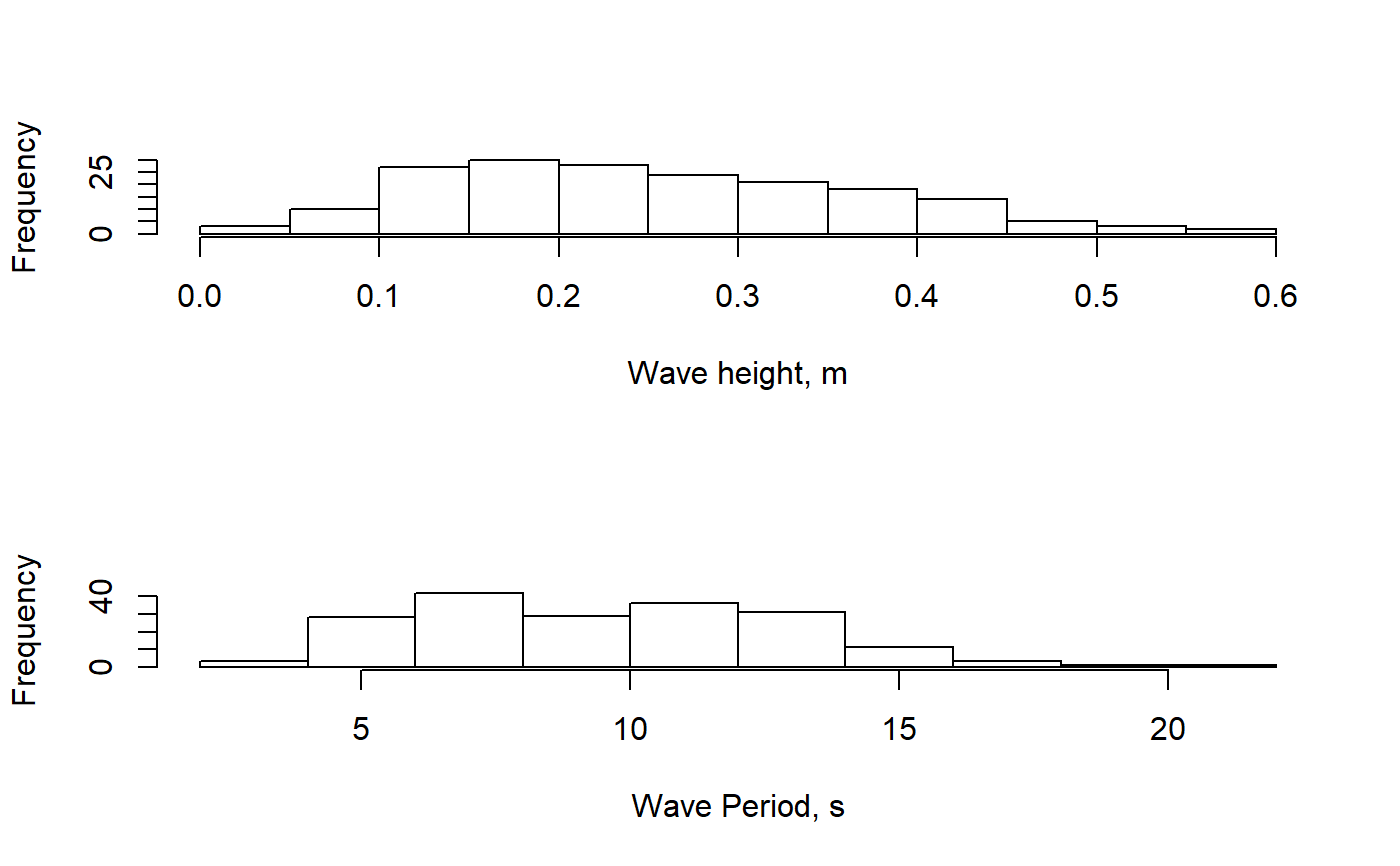Calculate ocean wave summary statistics, including significant wave height and period.

waveStatsZC(data, Fs, threshold = NULL, plot = FALSE)

## Arguments

data A numeric vector of water surface height data. The data do not need to be detrended prior to use. Typical units = meters Sampling frequency of the data, in Hz. The minimum height necessary for a zero-crossing event to be considered a wave. Set to TRUE if summary histograms of wave heights and wave periods are desired.

## Value

A list object containing summary statistic values.

• Hsig Mean of the highest 1/3 of waves in the data set. Units = same as input surface heights.

• Hmean Overall mean wave height, for all waves (bigger than threshold).

• H10 Mean height of the upper 10% of all waves.

• Hmax Maximum wave height in the input data.

• Tmean Mean period of all waves (bigger than threshold). Units = seconds.

• Tsig Mean period of Hsig (highest 1/3 of waves).

## Details

Based on an upward zero-crossing algorithm originally provided by Urs Neumeier, v1.06. However, by default the algorithm is run as a downward zero-crossing process by inverting the data.

Original MATLAB function by Urs Neumeier: http://neumeier.perso.ch/matlab/waves.html

waveStatsSP for wave statistics determined using spectral analysis methods.

## Examples

data(wavedata)
waveStatsZC(data = wavedata$swDepth.m, Fs = 4, plot = TRUE)#>$Hsig
#>  0.3870003
#>
#> $Hmean #>  0.2530191 #> #>$H10
#>  0.4686878
#>
#> $Hmax #>  0.565325 #> #>$Tmean
#>  9.622973
#>
#> \$Tsig
#>  11.81855
#>